If you wish to contribute or participate in the discussions about articles you are invited to contact the Editor

# Baseband Processing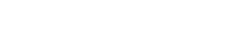Receivers
Title Baseband Processing
Edited by GMV
Year of Publication 2011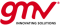The baseband processing block is responsible for processing the down-converted and digitized GNSS signal in order to provide observables: code pseudo-ranges and carrier phase measurements, as well as navigation data. Additional information, such as Doppler frequency, Carrier-to-Noise ratio, or lock indicators, can also be provided.

In most GNSS receivers’ architectures, the baseband processing relies on independent channels that track each satellite signal autonomously. Then, the information from each channel is integrated to derive a navigation solution.

## Block Diagram

The baseband processing block is usually replicated over several channels. Each channel processes a given signal from a given satellite in order to provide GNSS observables and navigation data. A generic diagram of a single channel within the baseband processing block is depicted in the following figure.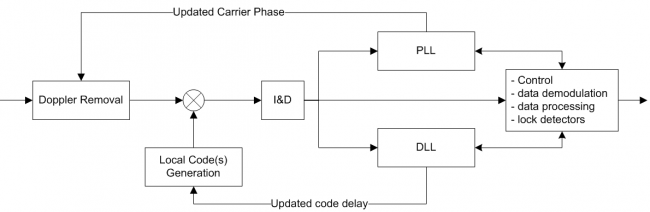Figure 1: Generic diagram of a single channel in the Baseband Processing block.

The incoming signal is first stripped of its Doppler frequency (according to the current estimation), and then correlated with one (or more) PRN code replicas generated locally (according to the current estimation of code delay). Then new estimations of the Doppler frequency and code delay are computed based on the assessment of the correlation outputs. Note that for GNSS using FDMA modulation schemes, such as GLONASS, the Doppler removal block represents a shift in the frequency, not only corresponding to the estimated Doppler shift caused by the relative motion between the satellite and the user, but also to the centre frequency of the satellite signal at hand.

This process is implemented in an iterative way, usually using PLL and DLL tracking loops to track the incoming signal's phase and code delay, respectively. In parallel, the receiver extracts the navigation data of the incoming signal, and ensures monitoring and control features further described here.

## Principle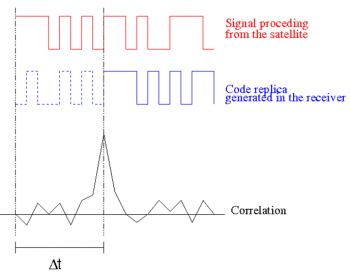Figure 2: The correlation process: the receiver shifts the code replica (by changing $\displaystyle{ \Delta t\, }$) until it finds a peak in the correlation output.

The basic principle of GNSS baseband processing relies on the correlation process. The underlying idea is that GNSS signals convey ranging codes that are built in a way that:

• When the code is correlated with an aligned replica of itself, the correlation output is maximum: high auto-correlation properties.
• When the code is correlated with a non-aligned replica of itself, the correlation output is low.
• When the code is correlated with another code of the same family, the correlation output is low: low cross-correlation properties.

The basic principle is illustrated in Figure 2. The receiver first assigns each channel with a PRN code: for GNSS based on CDMA (such as GPS and Galileo), each satellite transmits a dedicated PRN code, whereas for GNSS based on FDMA (such as GLONASS), the PRN code is the same for all satellites.

At channel level, the incoming signal is correlated with the local replica of this PRN code over time. This local replica is generated in such a way that its code delay and phase characteristics vary, representing a two-dimensional search over code and (Doppler) frequency. Whenever the local replica parameters (code and frequency) match those of the incoming signal, the correlation output will reach a maximum, and the receiver will consider this pair (code and frequency) as the current estimates for these parameters.

Real-life systems are very noisy and dynamic: as a consequence, the auto-correlation peak seems to fluctuate at each time instant. This fact justifies the need for tracking loops (accumulators and filters) to continuously “follow” the incoming signal, since an error of 1 ms in the code delay would lead to an error of around 300 km in the pseudorange measurement.

## Mathematical Model

At the output of the RF section, the baseband signal can be written as (neglecting noise):

$\displaystyle{ s_{BB} (t_k) = \Re\{s(t_k) exp[j\phi (t_k)]\}\, }$

with $\displaystyle{ s(t_k) = A_Im_I(t_k)d(t_k)-jA_Qm_Q(t_k)d(t_k)\, }$

where:

• $\displaystyle{ A\, }$ is the signal amplitude.
• $\displaystyle{ m(t_k)\, }$ = ±1, and it includes the PRN code and modulation information (e.g. subcarrier code for BOC modulations). For the case of GPS L1, $\displaystyle{ m_I(t_k)\, }$ and $\displaystyle{ m_Q(t_k)\, }$ are the C/A code and the P code respectively.
• $\displaystyle{ d(t_k)\, }$ is the navigation data. Note that if no data is transmitted (e.g. pilot channels), this term is always 1.
• $\displaystyle{ I\, }$ and $\displaystyle{ Q\, }$ refer to the In-phase and the Quadrature components, respectively.
• $\displaystyle{ \phi (t_k)\, }$ accounts for the phase information, including Doppler frequency, receiver clock instabilities and initial signal phase.

The Doppler removal block rotates the complex baseband signal by the current estimation of the carrier phase:

$\displaystyle{ s_{out} (t_k) = s(t_k) exp[j\phi_e (t_k)]\}\, }$

where:

• the phase error is given by $\displaystyle{ \phi_e (t_k)=\hat{\phi}(t_k)-\phi(t_k)\, }$
• $\displaystyle{ \hat{\phi}(t_k)\, }$ is the phase estimated at the receiver.
• $\displaystyle{ \phi(t_k)\, }$ is the phase of the incoming signal.

The correlation between the incoming signal and the local (PRN) code replica corresponds to the correlators and accumulators blocks, and can be written as:

$\displaystyle{ Corr_{out}(\hat{\tau}) = \sum_T s_{out}^{*} (t_k) m(t_k-\hat{\tau})\, }$

where:

• $\displaystyle{ T\, }$ is the integration time, i.e. the accumulation interval.
• $\displaystyle{ \hat{\tau}\, }$ is the code delay estimated at the receiver.

As an example, the correlation output for a receiver tracking GPS L1 C/A code would be:

$\displaystyle{ Corr_{out}(\hat{\tau}) = \sum_T (A_Id(t_k)c_I(t_k) + jA_Qd(t_k)c_Q(t_k)) exp[-j\phi_e(t_k)] c_I(t_k - \hat{\tau})\, }$

where:

• $\displaystyle{ c_I\, }$ would stand for C/A code.
• $\displaystyle{ c_Q\, }$ would stand for the P code.
• $\displaystyle{ c_I(t_k - \hat{\tau})\, }$ is the local replica generated at the receiver.

Considering that the cross-correlation between C/A code and P code can be neglected, the expression can be simplified to:

$\displaystyle{ Corr_{out}(\hat{\tau}) = \sum_T A_Id(t_k)c_I(t_k) exp[-j\phi_e(t_k)] c_I(t_k - \hat{\tau})\, }$

Hence the output of the correlation and accumulation blocks can be written in its in-phase ($\displaystyle{ I\, }$) and quadrature ($\displaystyle{ Q\, }$) components as:

$\displaystyle{ I_P = A_I d_I R_{c_I} (\tau_e) cos(\phi_e)\, }$

$\displaystyle{ Q_P = -A_Id_IR_{c_I}(\tau_e)sin(\phi_e)\, }$

where:

• $\displaystyle{ R_{c_I}\, }$ is the auto-correlation of the $\displaystyle{ c_I\, }$ code.
• $\displaystyle{ P\, }$ stands for the Prompt replica, i.e. the replica generated at the receiver which is aligned with the incoming signal.
• $\displaystyle{ \tau_e\, }$ is the error of the code delay estimated at the receiver.
• $\displaystyle{ \phi_e\, }$ is the error of the carrier phase estimated at the receiver.

The correlation outputs are then fed to the tracking loops. Further information about GNSS receiver correlators can be found here.

## Signal Detection

In the previous sections it's assumed that a current estimate of the parameter pair {code delay, Doppler frequency} was already available, and therefore the receiver would generate the local PRN code replica using those parameter estimates. However, when a channel is first set up, these estimates are usually not available, and therefore each channel will launch a cold search for the signal. The channel is said to be in Acquisition mode when searching for signals, and then transits to Tracking mode once the signal is found. For further information on receiver operations, see here.

When in acquisition mode, each channel is looking for all possible pairs of {code delay, Doppler frequency}, by generating a set of possible local replicas, and correlating each of them with the incoming signal. The power of the correlation output is somehow a measure of how good (or how close to the real signal) the estimates of the code delay and carrier phase are. Nevertheless, the correlation outputs described up to now are all based on the assumption that the signals are not subject to noise: in fact, the signal is actually so noisy that a single shot decision process on the correlation results has a very low probability of detecting the presence of a signal.

The presence of a signal is assessed using the following decision statistic:

$\displaystyle{ z= \sum_{k=1}^M |Y(k)|^2\, }$

where:

• $\displaystyle{ M\, }$ is the number of non-coherent integrations.
• $\displaystyle{ Y\, }$ is the output of the correlation of the incoming signal with the local replica (complex) over the coherent integration time $\displaystyle{ T\, }$.
• $\displaystyle{ k\, }$ refers to the $\displaystyle{ k\, }$th coherent integration interval.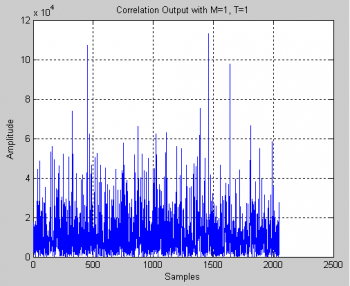Figure 3:Example of the correlation output for different code delays using 1 coherent and 1 non-coherent integration times.

The statistic $\displaystyle{ z\, }$ is then compared to a detection threshold, to assess whether the signal is present or not. This detection threshold is defined according to a target probability of false alarm.

In this process, the correlation outputs are actually integrated over time before further processing: this is gathered in the block I&D, standing for Integrate and Dump, in Figure 1. Two types of integration can be considered:

• Coherent integrations: this technique consists in using longer integration times (multiples of the code length) before dumping the correlation output. Although this technique reduces noise, its performance is limited by the bit boundaries that carry the navigation message: in fact, if the integration time is such that it straddles the bit boundaries, then the correlation output is bound to lose overall power.
• Non-coherent integrations: this technique consists in adding the results of single shot correlations together, before entering the decision process.

Due to squaring losses, the first technique is more effective that the latter, although limited by the navigation bit boundaries. As an example, Figure 3 represents the correlation outputs for all possible code delays in one single Doppler frequency (which we know beforehand to be the correct one). In this case, one coherent and one non-coherent integration were considered.

Because of the noisy conditions of GNSS signals, it is clear that the correlation peak is not visible from these results. To illustrate further, Figure 4 shows the correlation results for the same signal with different coherent (T) and non-coherent (M) integration times. The power increase becomes clear as M and T increase, hence showing clearly the correlation peak. The abscise value can be processed to yield a first estimate of the code delay.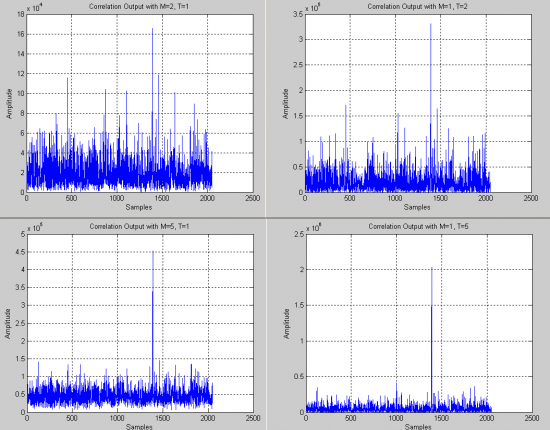Figure 4:Example of the correlation output for different code delays using different coherent and non-coherent integration times.

On one hand, the examples shown in Figure 4 illustrates how coherent integrations bring more benefits (higher power and lower noise floor) when compared to non-coherent integrations, which need to cope with squaring losses. On the other hand, non-coherent integrations are safer to implement (before bit synchronization), since they do not need to take into account the straddling navigation bit boundaries.

Finally, the dimensioning of these techniques has to consider the expected noise conditions, probability of false alarm, probability of detection and mean acquisition time. In fact, the longer the integrations the higher probability of detection (the lower the probability of false alarm) but the slower the potential acquisition time is, since the receiver will dwell longer in each search bin, defined by the pair [code delay, Doppler frequency].

The concepts of coherent and non-coherent integrations are also used when the receiver is in tracking mode, in order to reduce the noise levels and increase accuracy (see tracking loops for details). The advantage is that, after bit synchronization, the receiver may increase the integration time up to the bit duration (e.g. 20 ms for GPS L1 C/A). Modernized GNSS such as Galileo already have so-called pilot channels that do not convey any navigation data, and therefore can be used to further extend (coherent) integration times.

Long integration times are also used to track weak signals in harsh environments (such as indoors), but they are limited both by the data bit and by the accuracy of the clock/Doppler frequency estimation.

## Platforms

Most receivers implement the baseband processing algorithms in dedicated ASICs, DSPs or even FPGAs, as discussed here. The baseband processing block, however, may also be implemented in software, following a Software Defined Radio (SDR) philosophy. Although the software implementations provide a higher degree of flexibility, upgradeability and expandability, they still raise concerns regarding processing load and CPU power consumption in current commercial platforms.